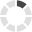## Change CountryLoading... Please wait...

#### Connect with us# Workplace Mathematics Series

## Product Description

This three-part training series will help students understand the fundamentals of elementary mathematics. The series builds practical skills step-by-step, beginning with whole numbers and ending with introductory algebra.

The Workplace Mathematics series is designed for those students who need to review or reinforce their grasp of elementary mathematics. To enter the programs and understand the content, students need only number recognition skills.

DVDs included:

Workplace Mathematics: Whole NumbersTeaches the decimal system of numbers, symbols, and formats for the representation of numbers in arithmetic.

Workplace Mathematics: FractionsTeaches how to work with fractions and decimals.

Workplace Mathematics: Introduction To AlgebraTeaches addition, subtraction, multiplication, and division of signed numbers, algebraic representations and terms, powers and exponents, and more.

Our DVDs are portable and easy-to-use. Training content is "chapterized," or organized by learning objectives to:

• Enable training administrators to cover specific training points and conduct refresher training
• Facilitate classroom discussion.

This four-part training series will help students understand the fundamentals of elementary mathematics. The series builds practical skills step-by-step, beginning with whole numbers and ending with introductory algebra.

The Workplace Mathematics series is designed for those students who need to review or reinforce their grasp of elementary mathematics. To enter the programs and understand the content, students need only number recognition skills.

eLearning courses included:

Workplace Mathematics: Whole NumbersTeaches the decimal system of numbers, symbols, and formats for the representation of numbers in arithmetic.

Workplace Mathematics: FractionsTeaches how to determine fractional parts of quantities, add and subtract fractions, and more.

Workplace Mathematics: DecimalsTeaches the basics of decimals, including place values, rounding, adding, subtracting, and more.

Workplace Mathematics: Introduction To AlgebraTeaches addition, subtraction, multiplication, and division of signed numbers, algebraic representations and terms, powers and exponents, and more.